R code

We will start with loading up the necessary packages using the `library()` command.

``library("tidyverse")``
``````## ── Attaching packages ─────────────────────────────────────── tidyverse 1.3.2 ──
## ✔ ggplot2 3.4.0      ✔ purrr   0.3.4
## ✔ tibble  3.1.8      ✔ dplyr   1.0.10
## ✔ tidyr   1.2.1      ✔ stringr 1.4.1
## ✔ readr   2.1.2      ✔ forcats 0.5.2
## ── Conflicts ────────────────────────────────────────── tidyverse_conflicts() ──

These slides utilize the ggplot2 graphics system to construct a number of graphical statistics, i.e. data visualizations, for data sets ranging from a single variable to a small number of continuous and categorical variables. The first step in constructing ggplot2 graphics is to organize your data in a `data.frame`.

# data.frame

Here we introduce the basics of `data.frame`s as extensions of matrices. As a reminder, matrices can only contain 1 data type in the entire matrix. In contrast, `data.frame`s can only contain 1 data type per column and thus make `data.frame`s an ideal storage unit for basic data sets.

Many data sets exist within the `datasets` package that is automatically installed when you install R.

``data()``

## ToothGrowth

We’ll take a look at the `ToothGrowth` data set. Since this data set is built into R, there is a helpfile with useful information about the data set.

``?ToothGrowth``

A variety of utility functions are available for assessing aspects of `data.frame` objects including

``dim(ToothGrowth)``
``##  60  3``
``head(ToothGrowth)``
``````##    len supp dose
## 1  4.2   VC  0.5
## 2 11.5   VC  0.5
## 3  7.3   VC  0.5
## 4  5.8   VC  0.5
## 5  6.4   VC  0.5
## 6 10.0   VC  0.5``````
``tail(ToothGrowth)``
``````##     len supp dose
## 55 24.8   OJ    2
## 56 30.9   OJ    2
## 57 26.4   OJ    2
## 58 27.3   OJ    2
## 59 29.4   OJ    2
## 60 23.0   OJ    2``````
``summary(ToothGrowth)``
``````##       len        supp         dose
##  Min.   : 4.20   OJ:30   Min.   :0.500
##  1st Qu.:13.07   VC:30   1st Qu.:0.500
##  Median :19.25           Median :1.000
##  Mean   :18.81           Mean   :1.167
##  3rd Qu.:25.27           3rd Qu.:2.000
##  Max.   :33.90           Max.   :2.000``````
``str(ToothGrowth)``
``````## 'data.frame':    60 obs. of  3 variables:
##  \$ len : num  4.2 11.5 7.3 5.8 6.4 10 11.2 11.2 5.2 7 ...
##  \$ supp: Factor w/ 2 levels "OJ","VC": 2 2 2 2 2 2 2 2 2 2 ...
##  \$ dose: num  0.5 0.5 0.5 0.5 0.5 0.5 0.5 0.5 0.5 0.5 ...``````

# ggplot2

As described in Section 3.2.3 of R4DS, the basic structure ggplot2 code is

``````ggplot(data = <DATA>) +
<GEOM_FUNCTION>(mapping = aes(<MAPPINGS>))``````

or

``````ggplot(data = <DATA>, mapping = aes(<MAPPINGS>)) +
<GEOM_FUNCTION>()``````

In both cases, you need to determine `<DATA>`, `<GEOM_FUNCTION>`, and `<MAPPINGS>`. In the former case, the mapping is only applied to that `<GEOM_FUNCTION>`. In the latter case, the mapping is applied to all future `<GEOM_FUNCTION>`s. I tend to default to the latter.

## Univariate plots

Let’s start by considering the response variable in this data set, `len`, i.e. the length of the odontoblasts.

### Histogram

ChatGPT: A histogram is a graphical representation of data distribution. It is a bar graph that represents the frequency of occurrence of continuous variables in the form of ranges or bins. The height of each bar represents the number of data points that fall within the range represented by that bin. Histograms are useful for analyzing the distribution of numerical data and identifying any patterns or trends in the data, such as skewness, outliers, and modality. They also help to visualize the shape of the distribution, which can be symmetrical, skewed, or multimodal. In addition, histograms are used in a wide range of applications, including image processing, signal processing, and statistical analysis.

``````ggplot(ToothGrowth, aes(x = len)) +
geom_histogram()``````
``## `stat_bin()` using `bins = 30`. Pick better value with `binwidth`.``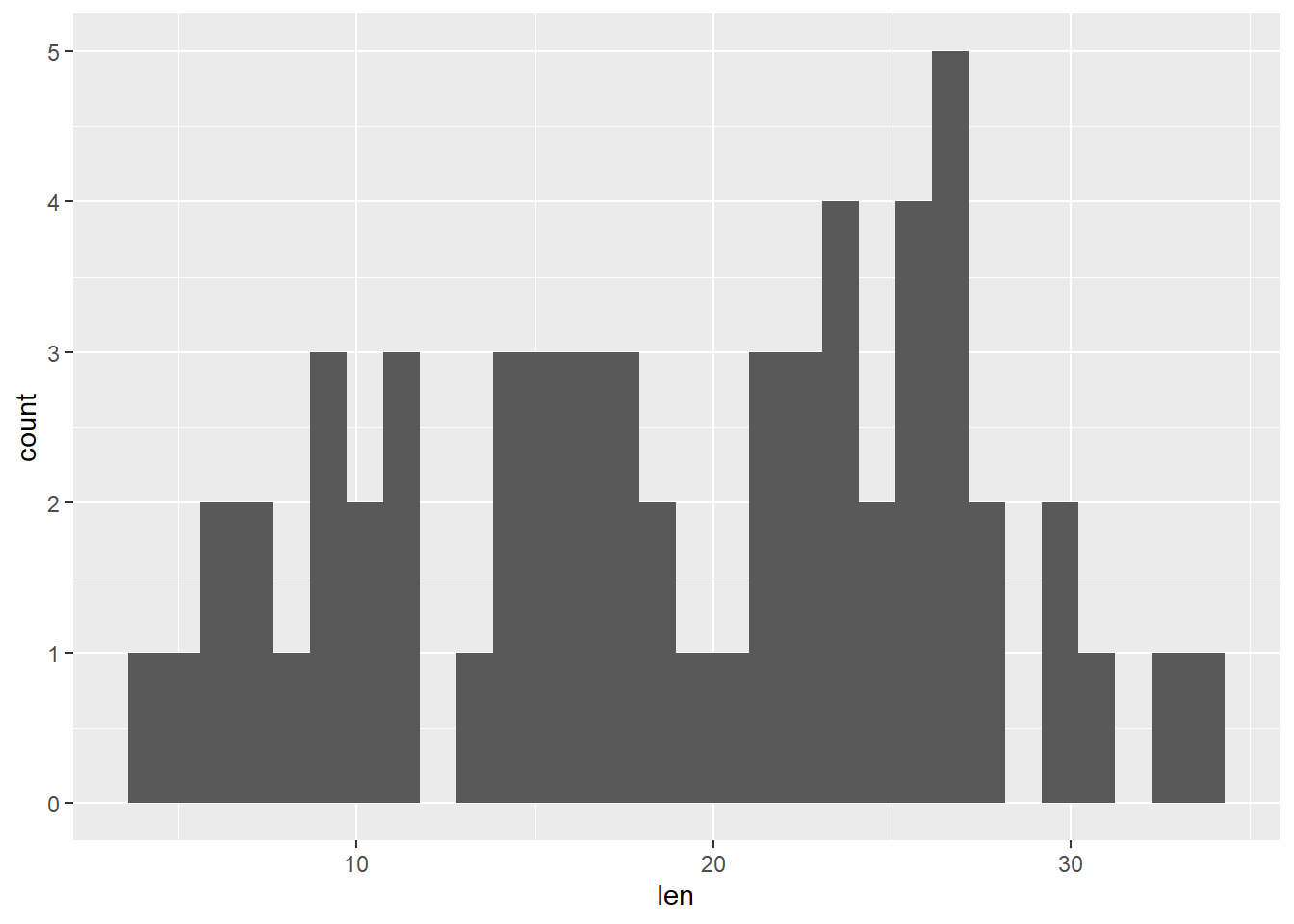As instructed, let’s choose the number of bins.

``````ggplot(ToothGrowth, aes(x = len)) +
geom_histogram(bins = 20)``````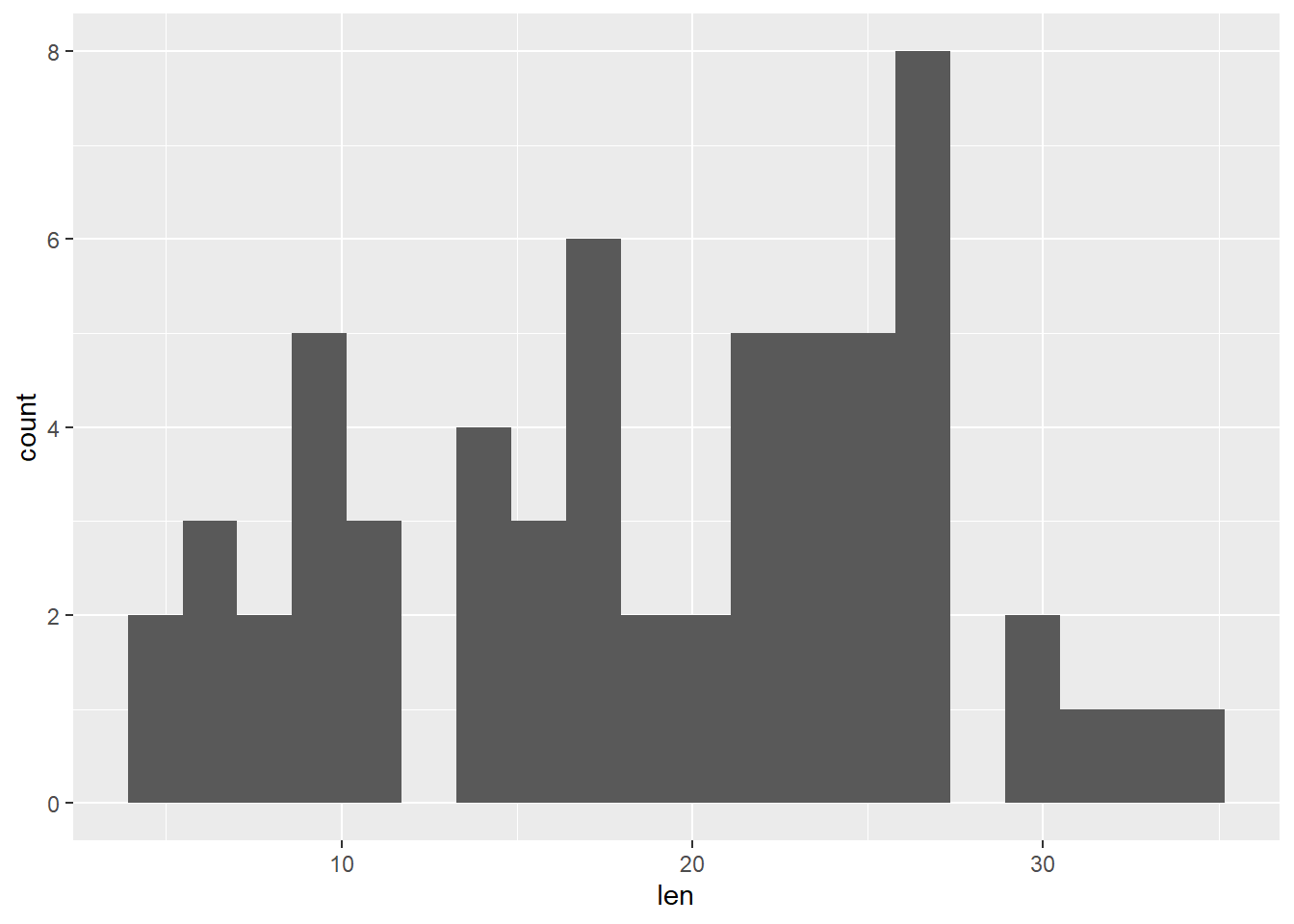Or set the binwidth (which is often more convenient)

``````ggplot(ToothGrowth, aes(x = len)) +
geom_histogram(binwidth = 1)``````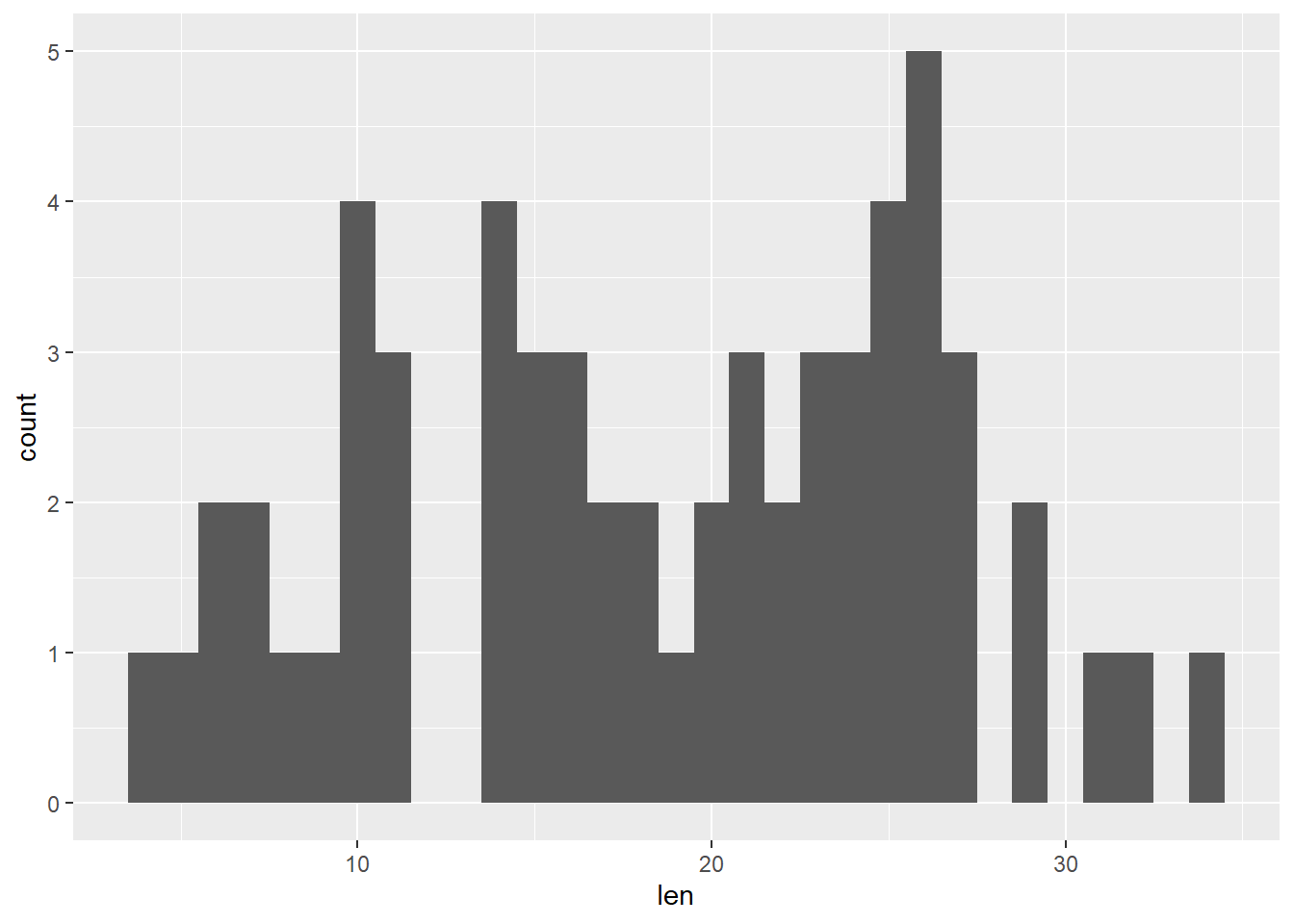If we would like to compare a histogram to a fitted probability density function, we may want to use proportion of counts rather than the number of counts, e.g.

``````ggplot(ToothGrowth, aes(x = len)) +
geom_histogram(aes(y = ..density..), binwidth = 1) +
stat_function(fun = dnorm,
args = list(mean = mean(ToothGrowth\$len),
sd   = sd(  ToothGrowth\$len)),
col = "red",
linewidth = 2)``````
``````## Warning: The dot-dot notation (`..density..`) was deprecated in ggplot2 3.4.0.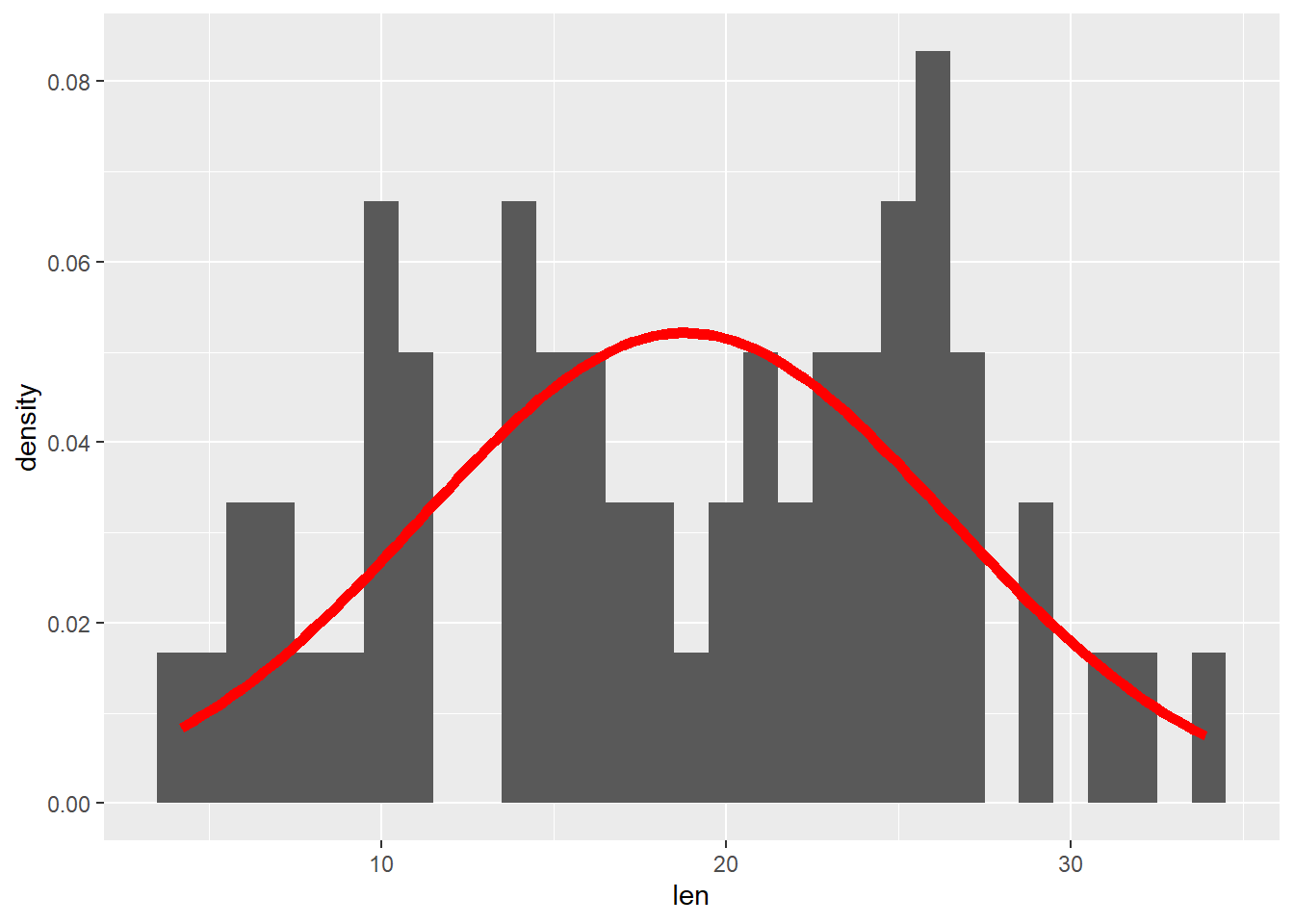### Boxplot

ChatGPT: A boxplot is a type of graph commonly used in statistics to represent and summarize the distribution of a set of numerical data. It is constructed by plotting a box between the first and third quartile (the 25th and 75th percentile), and a vertical line that extends from the box to the minimum and maximum value of the data (excluding outliers). The median (50th percentile) of the data is also plotted as a horizontal line inside the box. This graphical representation provides a quick visual summary of the central tendency, variability, and skewness of the data. Outliers, which are values that lie significantly outside the range of the majority of the data, are also easily identified in a boxplot.

``````ggplot(ToothGrowth, aes(x = len)) +
geom_boxplot()``````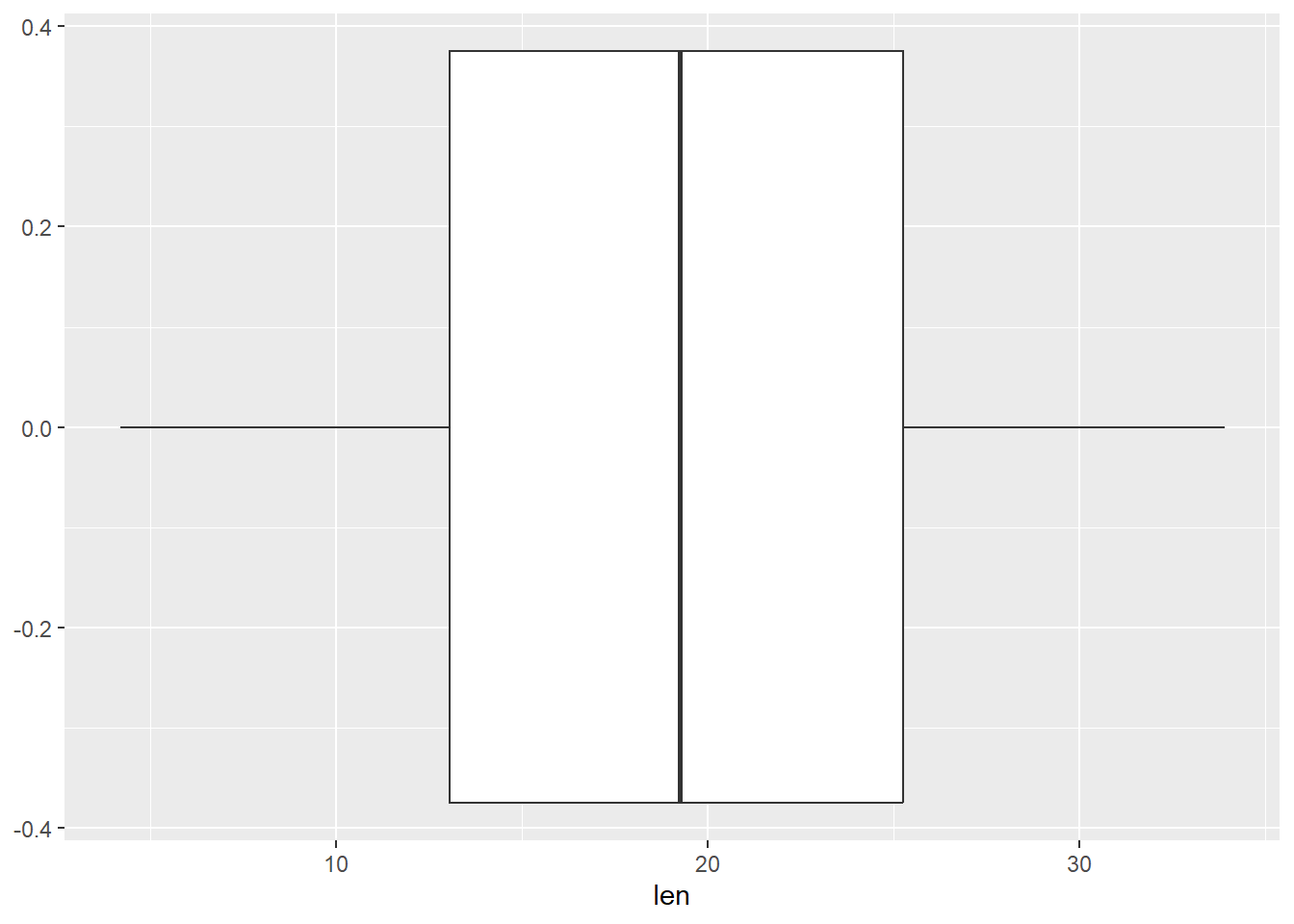### Density plot

``````ggplot(ToothGrowth, aes(x = len)) +
geom_density()``````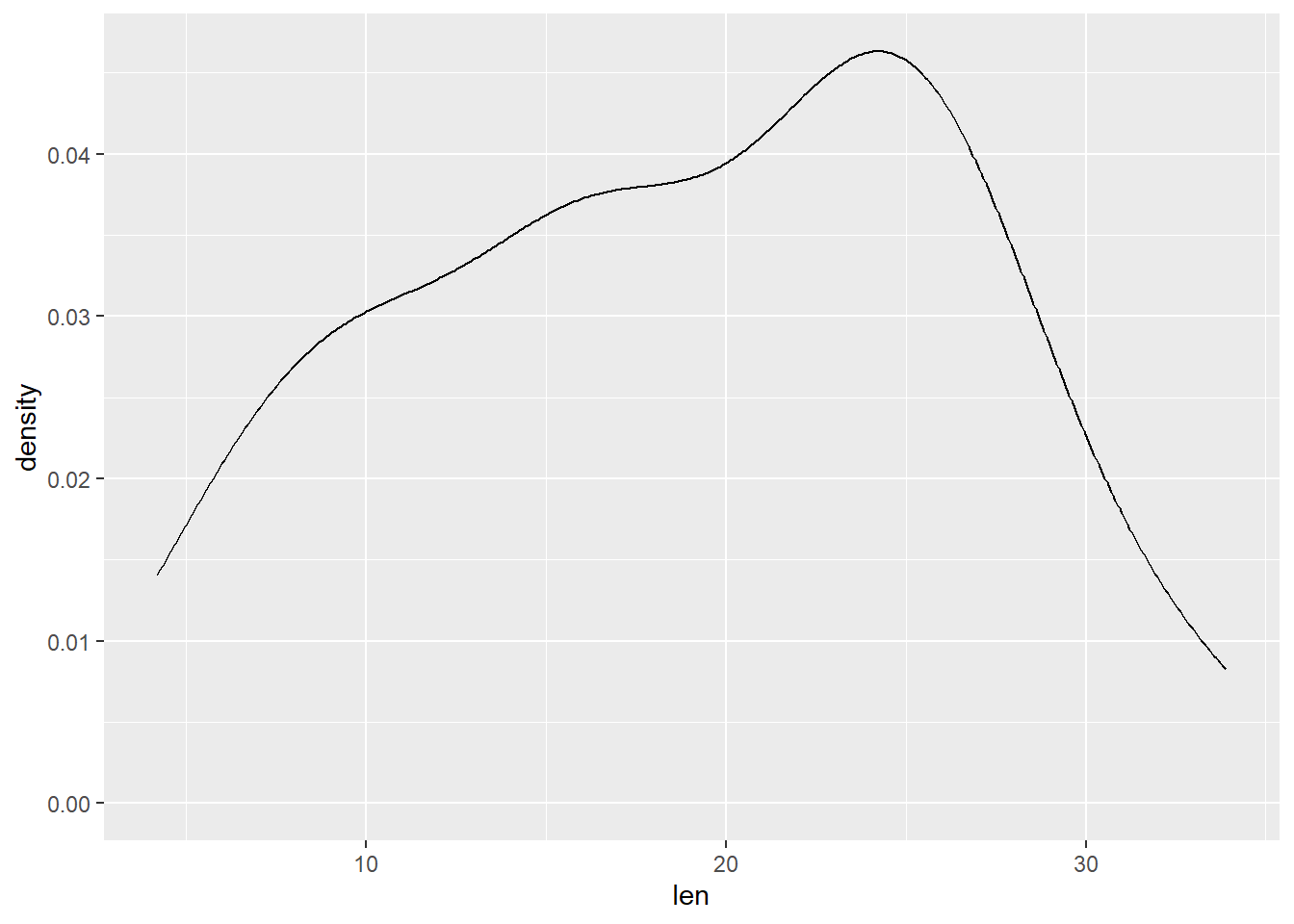### Violin plot

``````ggplot(ToothGrowth, aes(x = len, y = 1)) + # note the y = 1
geom_violin()``````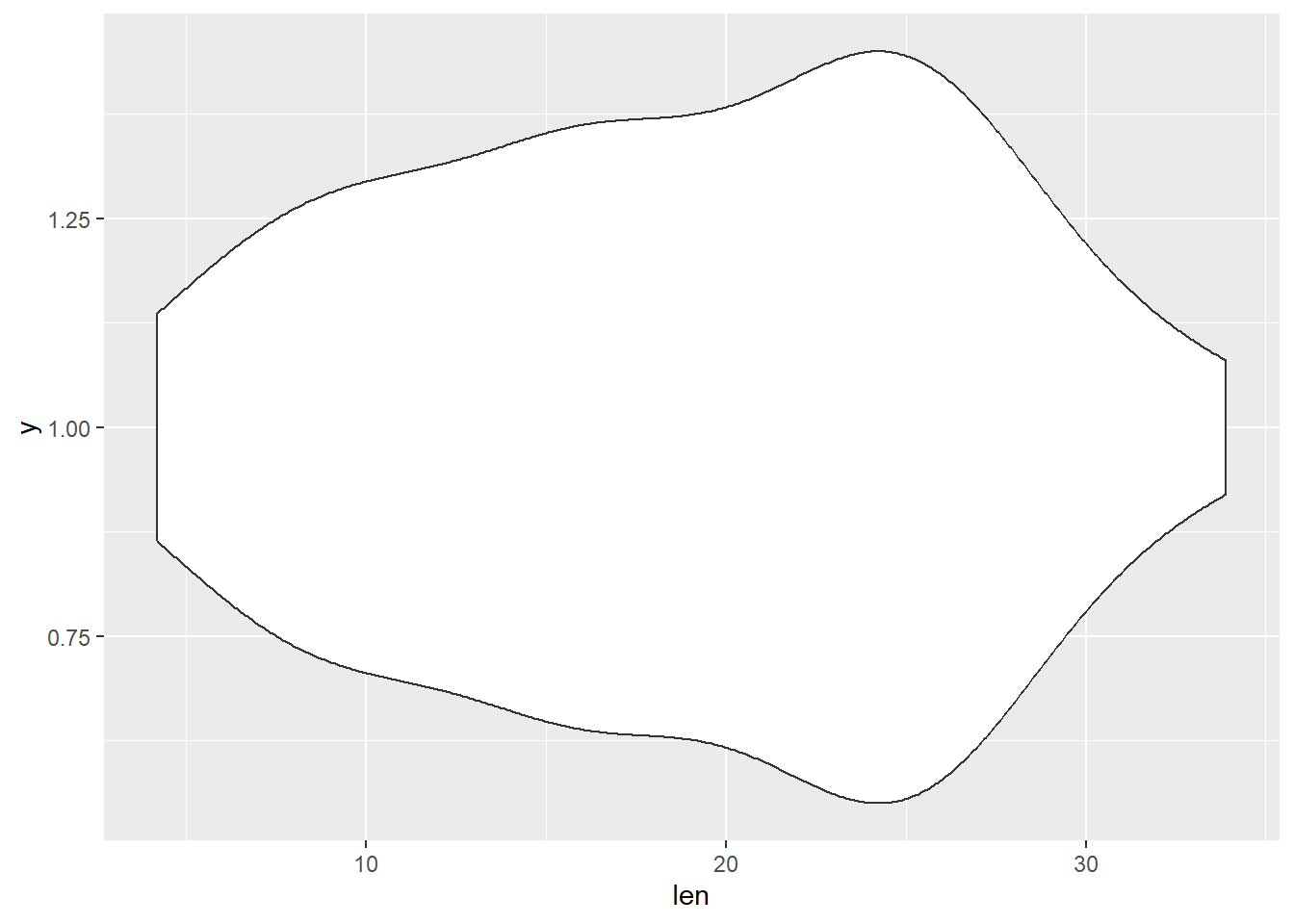## Multiple univariate plots

It is quite easy to construct multiple of these univariate plots according to categorical variables by adding in an additional aesthetic.

### Violin plots

``````ggplot(ToothGrowth, aes(x = len, y = supp)) +
geom_violin()``````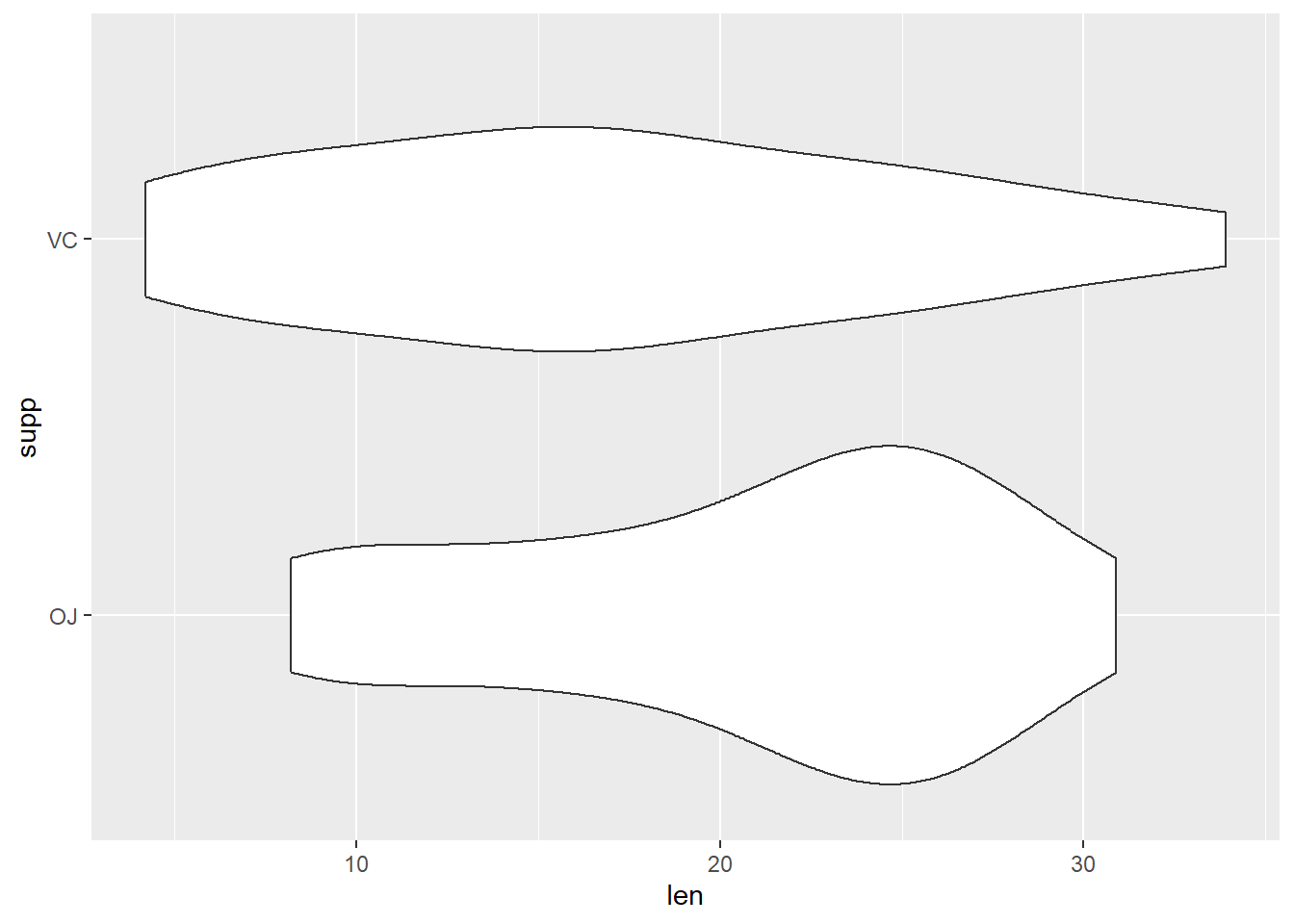``````ggplot(ToothGrowth, aes(x = len, y = supp)) +
geom_violin(trim = FALSE)``````